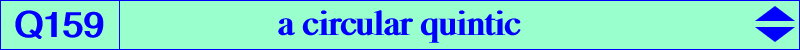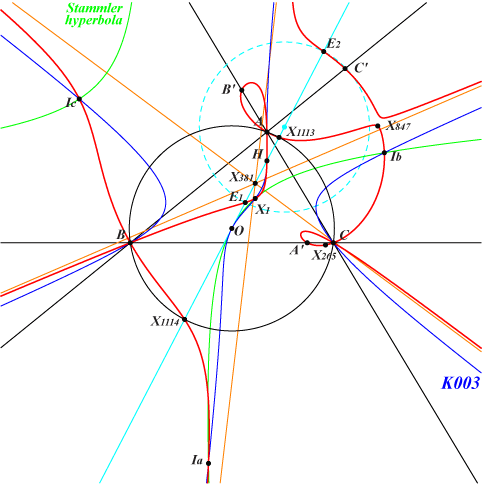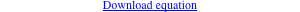too complicated to be written here. Click on the link to download a text file.X(1), X(4), X(265), X(847), X(1113), X(1114) excenters infinite points of K003 Ix-anticevian points, see Table 23 other points belowDenote by M' and M" the isogonal conjugates of M with respect to the anticevian and pedal triangles of M respectively. These three points are collinear if and only if M lies on Q159. The isogonal transform of Q159 is Q160. Q159 is a circular circum-quintic with two imaginary asymptotes passing through H and three real asymptotes parallel at X(381) to those of K003. Q159 is a member of the pencil of circular circum-quintics generated by the two following decomposed curves : union of K003 and the circum-circle, union of the line at infinity and the Stammler quartic Q066. Q159 meets the Euler line at X(4), X(1113), X(1114) and two other points E1, E2 on the circle with center X(3627) – on the Euler line – and radius √5/2 OH. The isogonal conjugates of these points lie on the line {578, 1199, 1204, etc}, obviously on the Jerabek hyperbola and on Q160. The isogonal transform of the tangent at A to the circle (CA) passing through A, X(3), X(186) meets BC at A' on Q159. B' and C' are defined likewise.